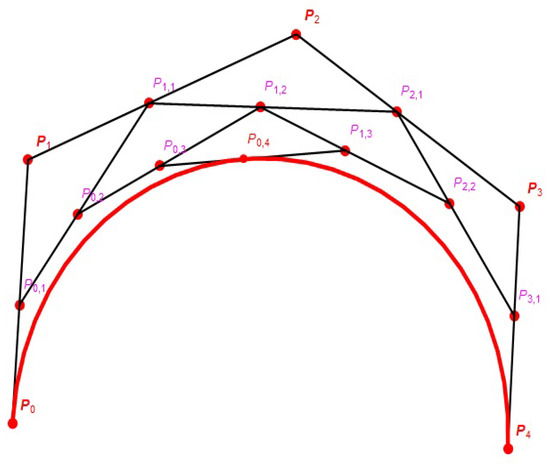Next Article in Journal
Coopetitive Games for Management of Marine Transportation Activity: A Study Case
Previous Article in Journal
Double Controlled Metric Type Spaces and Some Fixed Point Results

Article

# Serret-Frenet Frame and Curvatures of Bézier Curves

Department of Mathematics, Faculty of Arts and Sciences, Yildiz Technical University, 34220 Istanbul, Turkey
*
Author to whom correspondence should be addressed.
Mathematics 2018, 6(12), 321; https://doi.org/10.3390/math6120321
Received: 24 October 2018 / Revised: 24 November 2018 / Accepted: 5 December 2018 / Published: 12 December 2018
The aim of this study is to view the role of Bézier curves in both the Euclidean plane $E 2$ and Euclidean space $E 3$ with the help of the fundamental algorithm which is commonly used in Computer Science and Applied Mathematics and without this algorithm. The Serret-Frenet elements of non-unit speed curves in the Euclidean plane $E 2$ and Euclidean space $E 3$ are given by Gray et al. in 2016. We used these formulas to find Serret-Frenet elements of planar Bézier curve at the end points and for every parameter t. Moreover, we reconstruct these elements for a planar Bézier curve, which is defined by the help of the algorithm based on intermediate points. Finally, in the literature, the spatial Bézier curve only mentioned at the end points, so we improve these elements for all parameters t. Additionally, we calculate these elements for all parameters t using algorithm above mentioned for spatial Bézier curve. View Full-Text
Show FiguresFigure 1

MDPI and ACS Style

Erkan, E.; Yüce, S. Serret-Frenet Frame and Curvatures of Bézier Curves. Mathematics 2018, 6, 321. https://doi.org/10.3390/math6120321

AMA Style

Erkan E, Yüce S. Serret-Frenet Frame and Curvatures of Bézier Curves. Mathematics. 2018; 6(12):321. https://doi.org/10.3390/math6120321

Chicago/Turabian Style

Erkan, Esra, and Salim Yüce. 2018. "Serret-Frenet Frame and Curvatures of Bézier Curves" Mathematics 6, no. 12: 321. https://doi.org/10.3390/math6120321

Find Other Styles
Note that from the first issue of 2016, MDPI journals use article numbers instead of page numbers. See further details here.

1